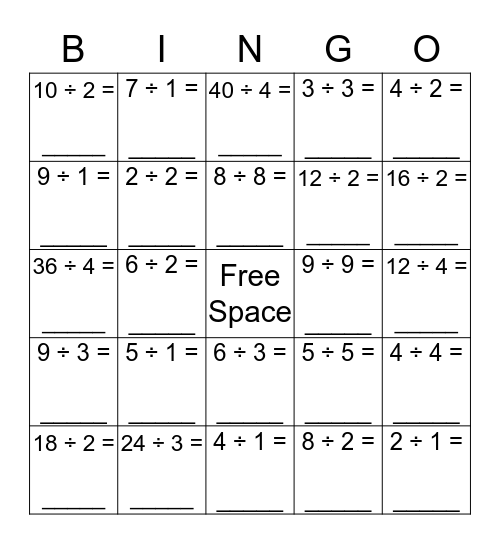# Division BINGOThis bingo card has a free space and 49 words: 1 ÷ 1 = _____, 2 ÷ 1 = _____, 3 ÷ 1 = _____, 4 ÷ 1 = _____, 5 ÷ 1 = _____, 6 ÷ 1 = _____, 7 ÷ 1 = _____, 8 ÷ 1 = _____, 9 ÷ 1 = _____, 10 ÷ 1 = _____, 2 ÷ 2 = _____, 3 ÷ 3 = _____, 4 ÷ 4 = _____, 5 ÷ 5 = _____, 6 ÷ 6 = _____, 7 ÷ 7 = _____, 8 ÷ 8 = _____, 9 ÷ 9 = _____, 10 ÷ 10 = _____, 4 ÷ 2 = _____, 6 ÷ 2 = _____, 8 ÷ 2 = _____, 10 ÷ 2 = _____, 12 ÷ 2 = _____, 14 ÷ 2 = _____, 16 ÷ 2 = _____, 18 ÷ 2 = _____, 20 ÷ 2 = _____, 6 ÷ 3 = _____, 9 ÷ 3 = _____, 12 ÷ 3 = _____, 15 ÷ 3 = _____, 18 ÷ 3 = _____, 21 ÷ 3 = _____, 24 ÷ 3 = _____, 27 ÷ 3 = _____, 30 ÷ 3 = _____, 8 ÷ 4 = _____, 12 ÷ 4 = _____, 16 ÷ 4 = _____, 20 ÷ 4 = _____, 24 ÷ 4 = _____, 28 ÷ 4 = _____, 32 ÷ 4 = _____, 36 ÷ 4 = _____, 40 ÷ 4 = _____, 10 ÷ 5 = _____, 15 ÷ 5 = _____ and 20 ÷ 5 = _____.

## Play Online